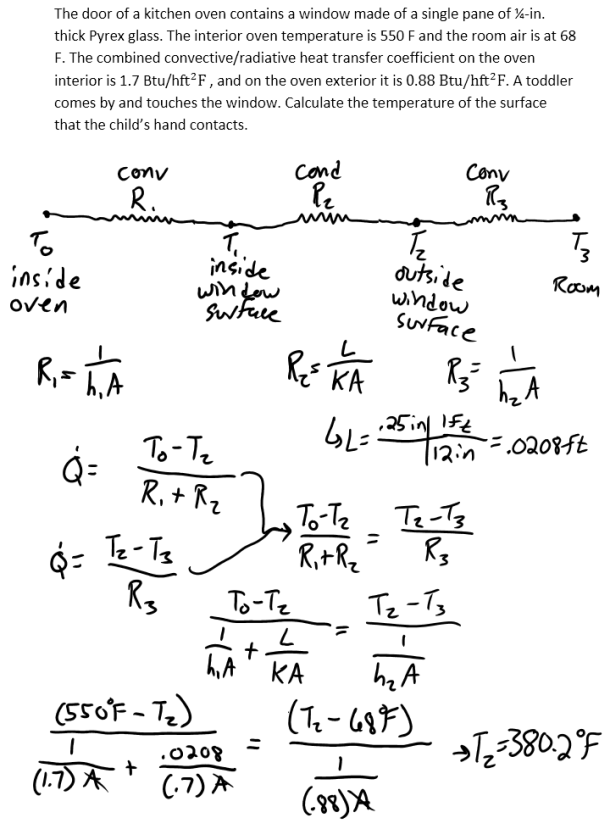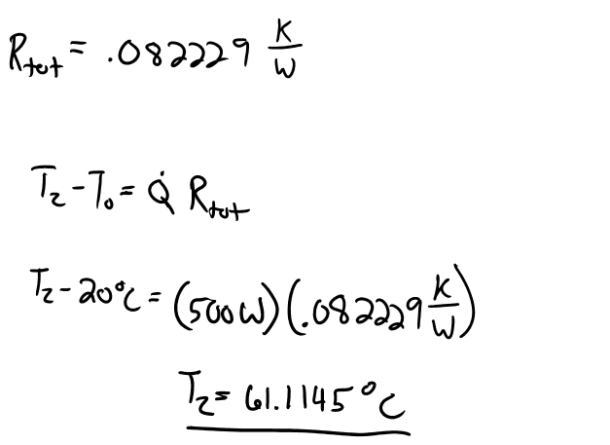heat and mass transfer problems engineering equations heat and mass transfer school homework engineering heat and mass transfer formulas heat and mass transfer solutions to heat and mass transfer problems full solution engineering problem solution heat and mass transfer math problems engineering equations heat and mass transfer school homework engineering solutions to heat and mass transfer formulas heat problems mass problem solutions to transfer problems full solution heat and mass transfer
heat and mass transfer problems engineering equations heat and mass transfer school homework engineering heat and mass transfer formulas heat and mass transfer solutions to heat and mass transfer problems full solution engineering problem solution heat and mass transfer math problems engineering equations heat and mass transfer school homework engineering solutions to heat and mass transfer formulas heat problems mass problem solutions to transfer problems full solution heat and mass transfer
Highalphabet Home Page heat and mass transfer problem solutions Heat and Mass Transfer Page
The door of a kitchen oven contains a window made of a single pane of Ľ-in. thick Pyrex glass. The interior oven temperature is 550 F and the room air is at 68 F. The combined convective/radiative heat transfer coefficient on the oven interior is 1.7 Btu/hft^2 F , and on the oven exterior it is 0.88 Btu/hft^2 F. A toddler comes by and touches the window. Calculate the temperature of the surface that the child’s hand contacts.The door of a kitchen oven contains a window made of a single pane of Ľ-in. thick Pyrex glass. The interior oven temperature is 550 F and the room air is at 68 F. The combined convective/radiative heat transfer coefficient on the oven interior is 1.7 Btu/hft^2 F , and on the oven exterior it is 0.88 Btu/hft^2 F. A toddler comes by and touches the window. Calculate the temperature of the surface that the child’s hand contacts.